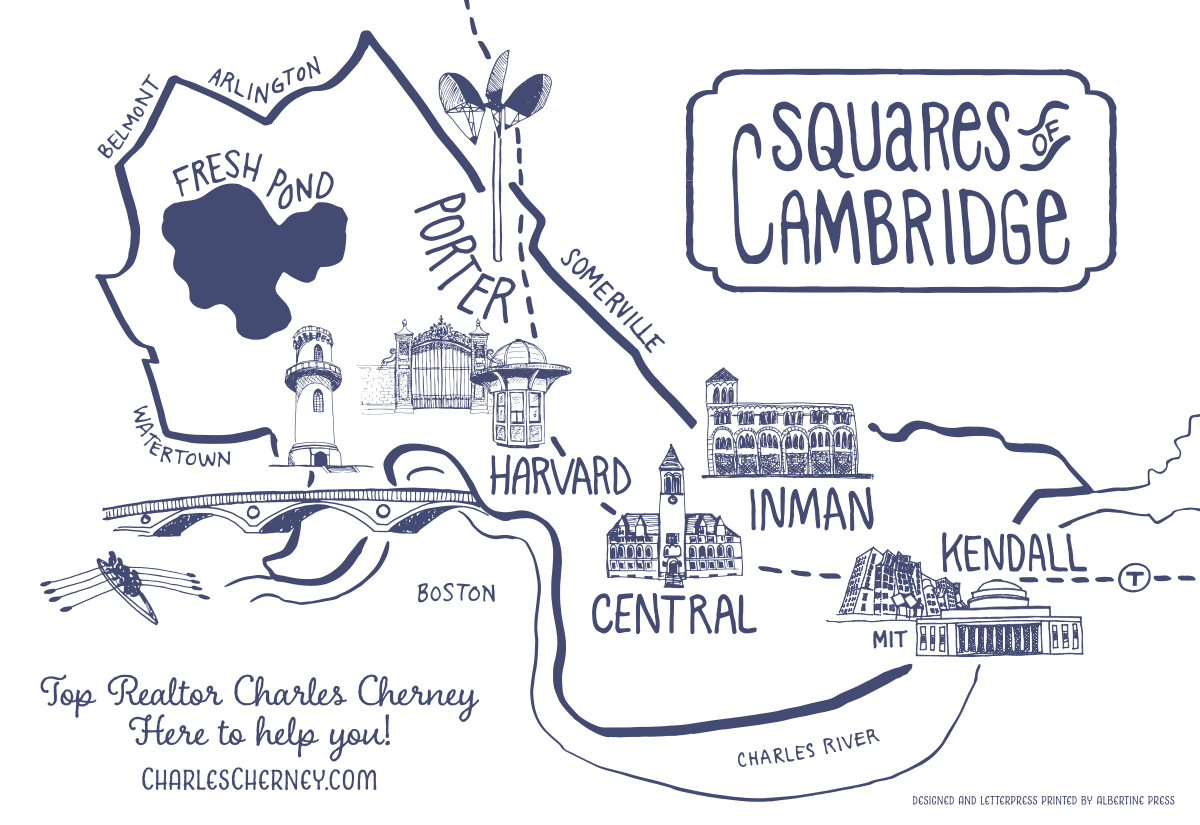Back To Blog

# What are the squares of Cambridge?What are the squares of Cambridge, Massachusetts?
The squares of Cambridge are
Porter Square, Harvard Square, Central Square, Inman Square and Kendall Square.

The interesting thing about the squares of Cambridge is that they are not square. At the heart of each square is a main intersection. In each instance, the square is defined as the area around the main intersection. The five main squares of Cambridge do not have clearly defined boundaries. The general area around the main intersection at the heart of the square defines the square.

You can think of each square as being located within a named neighborhood of Cambridge. So, then, Porter Square is in Neighborhood Nine; Harvard Square is in West Cambridge; Central Square is in the Port; Inman Square is in Wellington-Harrington; and Kendall Square is in East Cambridge. Check out my blog post about the named neighborhoods of Cambridge.

With the sole exception of Inman Square, each one of the squares has a Red Line subway station.

I have created an online magazine about each of the five squares of Cambridge.

You can check out these online magazines here:

Porter Square

Harvard Square

Central Square

Inman Square

Kendall Square

Call me at 617-733-8937 or email me.